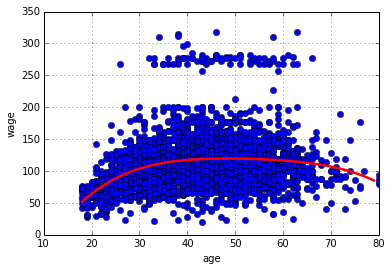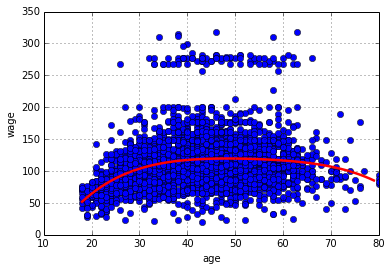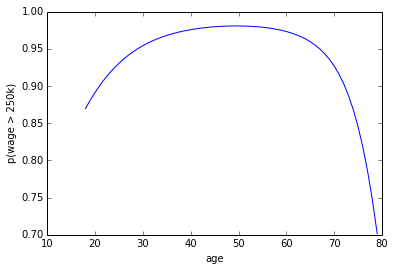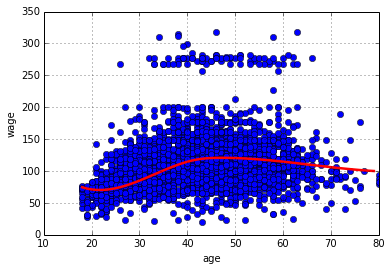Chapter 7: Nonlinear Models¶

In :
from __future__ import division
import pandas as pd
import numpy as np
import scipy as sp
import matplotlib.pyplot as plt
from sklearn.linear_model import LinearRegression
from sklearn.linear_model import LogisticRegression
from sklearn.metrics import mean_squared_error
from sklearn.feature_selection import SelectKBest
from sklearn.feature_selection import f_regression
from sklearn.feature_selection import chi2
from sklearn.cluster import MeanShift
from scipy.interpolate import LSQUnivariateSpline
%matplotlib inline
In :
# Data comes from ISLR::Wage - written using write.csv(Wage, "wage.csv", row.names=FALSE)
Out:
year age sex maritl race education region jobclass health health_ins logwage wage
0 2006 18 1. Male 1. Never Married 1. White 1. < HS Grad 2. Middle Atlantic 1. Industrial 1. <=Good 2. No 4.318063 75.043154
1 2004 24 1. Male 1. Never Married 1. White 4. College Grad 2. Middle Atlantic 2. Information 2. >=Very Good 2. No 4.255273 70.476020
2 2003 45 1. Male 2. Married 1. White 3. Some College 2. Middle Atlantic 1. Industrial 1. <=Good 1. Yes 4.875061 130.982177
3 2003 43 1. Male 2. Married 3. Asian 4. College Grad 2. Middle Atlantic 2. Information 2. >=Very Good 1. Yes 5.041393 154.685293
4 2005 50 1. Male 4. Divorced 1. White 2. HS Grad 2. Middle Atlantic 2. Information 1. <=Good 1. Yes 4.318063 75.043154

5 rows × 12 columns

In :
# factorize non-numeric variables
wage_df["sex"] = pd.factorize(wage_df["sex"])
wage_df["maritl"] = pd.factorize(wage_df["maritl"])
wage_df["race"] = pd.factorize(wage_df["race"])
wage_df["education"] = pd.factorize(wage_df["education"])
wage_df["region"] = pd.factorize(wage_df["region"])
wage_df["health"] = pd.factorize(wage_df["health"])
wage_df["health_ins"] = pd.factorize(wage_df["health_ins"])
Out:
year age sex maritl race education region jobclass health health_ins logwage wage
0 2006 18 0 0 0 0 0 1. Industrial 0 0 4.318063 75.043154
1 2004 24 0 0 0 1 0 2. Information 1 0 4.255273 70.476020
2 2003 45 0 1 0 2 0 1. Industrial 0 1 4.875061 130.982177
3 2003 43 0 1 1 1 0 2. Information 1 1 5.041393 154.685293
4 2005 50 0 2 0 3 0 2. Information 0 1 4.318063 75.043154

5 rows × 12 columns

Polynomials¶

We attempt to build a model to predict wage given a degree 4 polynomial over age. In R the polynomial features are created using the poly() function. As pointed out in this post from Dave Moore the method creates orthogonal polynomial features, which are different from simple powers of the original feature. Code for the poly() function shown below is a straight copy from the ortho_poly_fit() function code from this page.

In :
def poly(x, degree = 1):
n = degree + 1
x = np.asarray(x).flatten()
if(degree >= len(np.unique(x))):
print("'degree' must be less than number of unique points")
return
xbar = np.mean(x)
x = x - xbar
X = np.fliplr(np.vander(x, n))
q, r = np.linalg.qr(X)

z = np.diag(np.diag(r))
raw = np.dot(q, z)

norm2 = np.sum(raw ** 2, axis=0)
alpha = (np.sum((raw ** 2) * np.reshape(x,(-1,1)), axis=0) / norm2 + xbar)[:degree]
Z = raw / np.sqrt(norm2)
return Z, norm2, alpha

X = poly(wage_df["age"].values, 4)
X[0:5, 1:]
Out:
array([[-0.0386248 ,  0.05590873, -0.07174058,  0.08672985],
[-0.0291326 ,  0.02629807, -0.01454995, -0.00259928],
[ 0.00409008, -0.01450655, -0.00013318,  0.01448009],
[ 0.00092602, -0.0148314 ,  0.00451367,  0.01265751],
[ 0.01200024, -0.00981585, -0.01113663,  0.01021146]])
In :
y = wage_df["wage"].values
reg = LinearRegression()
reg.fit(X, y)
print "Intercepts:", reg.intercept_
print "Coefficients:", reg.coef_
ax = wage_df.plot(x="age", y="wage", style="o")
ax.set_ylabel("wage")
# The poly() method cannot return features for a single X value, so we have
# to plot the raw predictions.
age = wage_df["age"].values
ypred = reg.predict(X)
polyline = np.poly1d(np.polyfit(age, ypred, 4))
xs = range(int(np.min(age)), int(np.max(age)))
ys = polyline(xs)
ax.plot(xs, ys, 'r', linewidth=2.5)
Intercepts: -6287691072.04
Coefficients: [  3.44391030e+11   4.47067854e+02  -4.78315807e+02   1.25521688e+02
-7.79111830e+01]
Out:
[<matplotlib.lines.Line2D at 0x3403850>]We can repeat this experiment with manually calculated powers of the age feature as follows. Even though the parameters are different, the resulting linear model appears to be the same.

In :
x1 = wage_df["age"].values
x2 = np.power(x1, 2)
x3 = np.power(x1, 3)
x4 = np.power(x1, 4)
X = np.vstack((x1, x2, x3, x4)).T
X[0:5, :]
Out:
array([[     18,     324,    5832,  104976],
[     24,     576,   13824,  331776],
[     45,    2025,   91125, 4100625],
[     43,    1849,   79507, 3418801],
[     50,    2500,  125000, 6250000]])
In :
y = wage_df["wage"].values
reg = LinearRegression()
reg.fit(X, y)
print "Intercepts:", reg.intercept_
print "Coefficients:", reg.coef_
ax = wage_df.plot(x="age", y="wage", style="o")
ax.set_ylabel("wage")
xs = range(int(np.min(x1)), int(np.max(x1)))
ys = [reg.predict([x, x**2, x**3, x**4]) for x in xs]
ax.plot(xs, ys, 'r', linewidth=2.5)
Intercepts: -184.154179774
Coefficients: [  2.12455205e+01  -5.63859313e-01   6.81068771e-03  -3.20383037e-05]
Out:
[<matplotlib.lines.Line2D at 0x5d2d8d0>]R's summary() function applied to a linear model returns p-values for each coefficient, which enables one to guage the significance of each feature. Scikit-Learn does not provide anything similar, but it does provide methods for feature selection which provides p-values and scores. Based on p-values and scores below, it appears that the 4th degree feature is the least important of the four. However, R reports a p-value of 0.05 for the 4th power.

In :
selector = SelectKBest(score_func=f_regression, k=10)
selector.fit(X, y)
(selector.scores_, selector.pvalues_)
Out:
(array([ 119.31172277,   77.71495649,   46.35938463,   25.11432639]),
array([  2.90077768e-27,   1.97798773e-18,   1.18437983e-11,
5.71655064e-07]))

The p-values observed from a linear model's summary() output only works as an indicator of feature importance if a single feature is being used. For multiple features, R advises the use of ANOVA. It turns out that the SelectKBest feature selector is based on ANOVA. The R example manually built models based on different feature sets then applied ANOVA to the models - there is no direct analog to that here.

Polynomial Logistic Regression¶

Fitting a Logistic Regression model to a binary response variable. The response variable is "high-earners" where the salary > 250K.

In :
X = np.vstack((x1, x2, x3)).T
y = wage_df["wage"].map(lambda w: 1 if w > 250 else 0).values
reg = LogisticRegression()
reg.fit(X, y)
print "Intercepts:", reg.intercept_
print "Coefficients:", reg.coef_
ypred = reg.predict(X)
print "MSE:", mean_squared_error(y, ypred)

# Plot the predicted probability of being a high-earner over age range
ages = wage_df["age"].values
xs = range(np.min(ages), np.max(ages))
ys = [reg.predict_proba(np.array([x, x*x, x*x*x])) for x in xs]
plt.plot(xs, ys)
plt.xlabel("age")
plt.ylabel("p(wage > 250k)")
Intercepts: [-0.00721825]
Coefficients: [[ -9.77916678e-02  -8.45456184e-04   2.46527805e-05]]
MSE: 0.0263333333333
Out:
<matplotlib.text.Text at 0x6075b10>Splines¶

Splines is a curve approximated by a series of straight lines. The breaks in the straight lines are called knots. The idea is to fit multiple linear models to these ranges, one each for each straight line segment. Scipy offers the UnivariateSpline but it requires manual knot selection. The code snippet below uses Mean-Shift clustering to automatically derive the knots based on this thread on StackOverflow.

In :
ages = wage_df["age"].values
wages = wage_df["wage"].values
X = np.vstack((ages, wages)).T
# cluster points to find the knot location
msc = MeanShift()
msc.fit(X)
knots = msc.cluster_centers_[:, 0]

# fit a spline over the points
spl = LSQUnivariateSpline(ages, wages, knots)
xs = range(np.min(ages), np.max(ages))
ys = spl(xs)

# plot the points and the spline
ax = wage_df.plot(x="age", y="wage", style="o")
ax.set_ylabel("wage")
ax.plot(xs, ys, 'r', linewidth=2.5)
Out:
[<matplotlib.lines.Line2D at 0x3403210>]